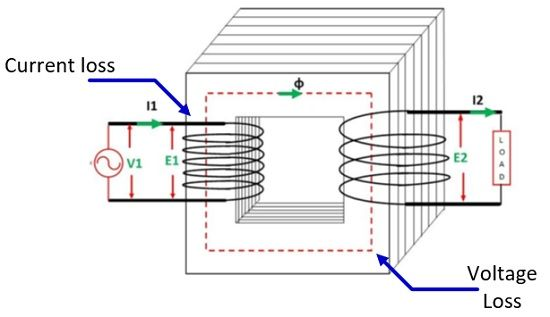# Rating of Transformer in kVA

The rating is the load carrying capability of the machine. It shows the maximum value of the voltage at which the machine is designed, and the current consumption occurs at that voltage. The machines are always rated in watts. But the alternator and the transformer are the only machines which are rated in volt-amp (VA).

## Why transformer is rated in KVA?

The machines which consumed power are rated in KW. But the main work of transformer is to transmit the power and not to consume the power, i.e. it is rated in VA and not in KW.

There are two reasons because of which the rating of the transformer is in kVA. Let understand this in details.

Reason 1: Before going to the topic first understand what is the difference between the KVA and KW.

The KVA is the product of the KV and ampere,The KW is the product of KV, Ampere and power factor,The above equations show that the power factor is the only terms which make the difference between the two.

### What is power factor?

The power factor simply shows the total power consumes by the load. i.e., The machines which are rated in KW are designed for the fixed type of load. Like inductive load are always connected to the induction machines.

But here in case of the transformer, the load is completely unpredictable, and the designer designed the transformer by considering the unity power factor. Thereby, the rating of the transformer is in KVA and not in KW. The rating of the transformer also expressed in VA, KVA and MVA depending on the size of transformer.

Reason 2: The transformer consumes the power regarding losses. The copper and iron are the two type of losses occur in the transformer. The copper loss depends on the current (ampere) flows through the windings of the transformer while the iron loss depends on the voltage (volts). i.e., the rating of the transformer is in kVA.How rating of transformer depends on their losses?

The rating of transformer depends on the temperature of their windings and oil that reaches within the specified limit. But how the temperature rises in the machine. It rises because of the losses. The losses that occur in the transformer increases their temperature. Thus, we can say that the rating of the transformer is in KVA.